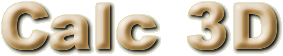## FAQ• What algorithm do you use to calculate the shortest distance between two points?

You have two points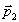The distance is=.

• What algorithm do you use to calculate the point on a line (footpoint), that is closest to another point, that is not on the line?
 You have a pointand a line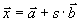;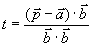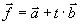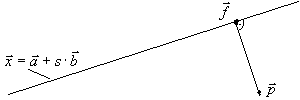• What algorithm do you use to calculate the shortest distance between a point and a line?

I calculate the distance between the Point and the Footpoint. (see Question before)

• What algorithm do you use to calculate the shortest distance between two lines?

You have 2 lines:,Ifthen calculate the distance between the point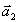and the line.

Else calculate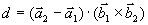if not (d=0 or h=0) then the distance is d/h.

• What algorithm do you use to calculate the distance between two vectors?

This question confuses the words "vector" and "point". A vector has a direction and a length. A point can be describted with a vector relative to another point. If I write only a vector for a point, the vector describts the point relative to the zeropoint. A distance between two vectors doesn’t make sense. It can be compared with the distance between left and right.

• What algorithm do you use to calculate the point between two points?